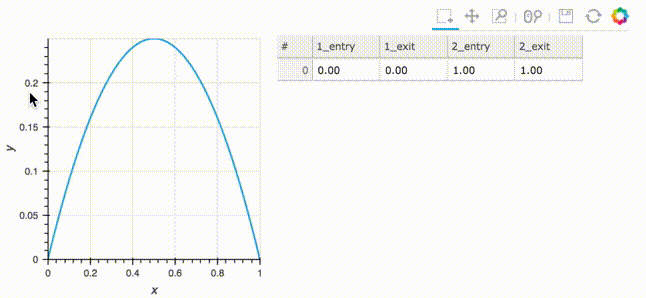# BoundsY#

## Title: BoundsY stream example#

Description: A linked streams example demonstrating how to use BoundsY streams.

Dependencies: Bokeh

Backends: Bokeh, Plotly

```import numpy as np
import holoviews as hv
from holoviews import opts, streams

hv.extension('bokeh')
``````xs = np.linspace(0, 1, 200)
ys = xs*(1-xs)
curve = hv.Curve((xs,ys))

bounds_stream = streams.BoundsY(source=curve,boundsy=(0,0))

def make_area(boundsy):
return hv.Area((xs, np.minimum(ys, boundsy), np.minimum(ys, boundsy)), vdims=['min','max'])

def make_items(boundsy):
times = ["{0:.2f}".format(x) for x in sorted(np.roots([-1,1,-boundsy])) + sorted(np.roots([-1,1,-boundsy]))]
return hv.ItemTable(sorted(zip(['1_entry', '2_exit', '1_exit', '2_entry'], times)))

area_dmap = hv.DynamicMap(make_area, streams=[bounds_stream])
table_dmap = hv.DynamicMap(make_items, streams=[bounds_stream])

(curve * area_dmap + table_dmap).opts(
opts.Layout(merge_tools=False),
opts.Area(tools=['ybox_select'], active_tools=['ybox_select'])
)
```This web page was generated from a Jupyter notebook and not all interactivity will work on this website. Right click to download and run locally for full Python-backed interactivity.